# idelem

## 紫微代数 1

0 安十二宫 起寅宫，先顺数生月 (2) 到卯，再逆数生时 (12) 到辰。命宫的位置在辰。注意这一步计数是包括起点宫位的。0.5 安身宫 起寅宫，先顺数生月 (2) 到卯，再顺数生时 (12) 到寅。 易证：身宫只可能为命、夫、财、迁、官、福之一，因为它和命宫之间隔着双倍的生时。

1 起寅首 出生年为辛年 (8)，则寅宫宫干为 $(8*2+1) \mod 10 = 7$，为庚。

$x$ $(x * 2 + 1)\mod 10$ $x$ $(x * 2 + 1)\mod 10$
1 3 6 3
2 5 7 5
3 7 8 7
4 9 9 9
5 1 10 1

2 定纳音五行局 命宫为壬辰，于是查表，为水二局。 因为五行局是相邻的阴阳干、阴阳支为一组，每一年的寅宫宫干相同，而命盘中相邻时辰必有相邻的阴阳干支，故每天有六个局，且和前后连续。

3 定紫微星 这一步比较复杂，而且是第一个用到出生日的步骤。

2 3 26 27 10 11 18 19
4 5 28 29 12 13 20 21
6 7 30 14 15 22 23
8 9 16 17 1 24 25

3 5 7 15 17 19 27 29
6 8 10 18 20 22 30
1 9 11 13 21 23 25 23
4 12 14 16 24 26 2 28

4 7 13 10 20 23 29 26
8 11 17 14 28 27 1 30
2 12 15 21 18 28 5
6 16 19 25 22 3 9

5 9 17 1 13 25 29 21
10 14 22 6 18 30 2 26
3 15 19 27 11 23 7
8 20 24 16 28 4 12

6 11 21 2 16 30 7 26
12 17 27 8 22 3 13
4 18 23 14 28 9 19
10 24 29 1 20 5 15 25

def ziwei(day: int, wuxing: int) -> int:
m = day % wuxing
if m:
start = pow(-1, wuxing - m) * (wuxing - m)
else:
start = -1
step = 3 + start + day // wuxing
return step


4 定天府星 天府和紫微永远关于寅-申线对称。 从天文的角度来说，寅申是立春和立秋，而紫微天府分别代表北斗和南斗星系的主星。 设 a 为紫微地支，b 为天府地支，则它们符合 $(a + b) \mod 3 = 6$ 的关系。 因为关于寅-申对称，所以上一步逆时针数就会得到天府星。

5 定南北斗中天诸星

1. （逆时针）紫微-天机-空-太阳-武曲-天同-空-空-廉贞-空-空-空
2. （顺时针）天府-太阴-贪狼-巨门-天相-天梁-七杀-空-空-空-破军-空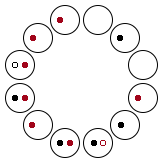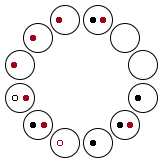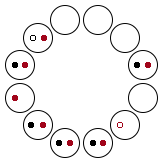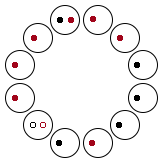1. 天府系的杀破狼永远在彼此的三方位置
2. 紫微系的紫武廉永远在彼此的三方位置
3. 紫微系诸星的对宫没有紫微系星 (因为紫微系星只有 6 颗，保证其序数 mod 6 不重复即可)
4. 天府对宫永远是七杀
5. 天相对宫永远是破军
6. 巨门不可能逢天府系星
7. 太阳不可能逢紫微系星
8. 剩下的星星里，天机-天同，天府-天相，太阴-天梁均成三合 (120°) 关系

6 安辅弼昌曲空劫 辰宫开始顺时针数生月为左辅，戌宫开始逆时针数生月为右弼。 辰宫开始顺时针数生时为文曲，戌宫开始逆时针数生时为文昌。 亥宫开始顺时针数生时为地劫，亥宫开始逆时针数生时为地空。

• 辅弼、昌曲都关于丑未线对称（辰戌丑未是四墓地）
• 空劫关于巳亥线对称
• 同时辰出生则昌曲、空劫位置相同
• 同月出生则辅弼位置相同
• 若要命三方四正同时逢昌曲，则需昌曲分别在卯亥、巳酉、未、丑、辰戌的位置，且满足一定生时条件。经计算，有下列几种情况：

• 若要命被辅弼夹，则需命在丑未：

• 地空文昌、地劫文曲不可能同宫

7 安魁钺

8 安禄存擎羊陀罗（年干）

• 如果在命盘上顺时针走，必会先后连续遇到陀罗、禄存、擎羊
• 四墓地没有禄存
• 四陷地没有陀罗
• 四马地没有擎羊（亦即，擎羊不在角上）

9 安天马（年支）

• 天马必在四马地（必不逢擎羊）

10 安火星铃星（年支+生时）

TBC

## Processing 笔记本 (2)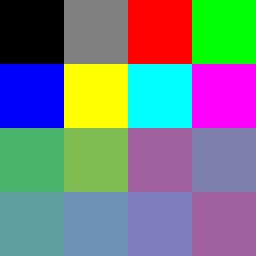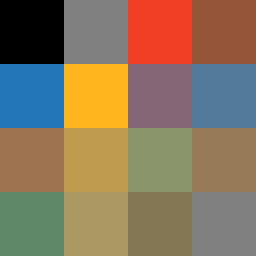• 类和对象
• 如何显示对象
• 从文件读取

### 需求和交互设计（打草稿！）

• 正方形，划分成 16 格，每格里有颜色或空白
• 左键混色（落笔），右键吸色（蘸取）
• 颜料转移时会被稀释，便于控制添加颜色的量（后来发现很难做，要重构）

### 程序框架

import java.util.*;
import java.text.*;

// 记录当前颜色和透明度的全局变量
color brush_color = color(0, 0, 0);
float brush_alpha = 0.0;
// TODO  我们还需要定义一个 Paint 类来储存和显示颜料，过会儿再说
// Paint[] palette = new Paint ;

// 这是启动时执行的函数，用于初始化一些数据
void setup() {
// 分辨率 256*256，每格宽高 64
size(256, 256);
// TODO 在这里初始化和显示所有颜料
}

// 每帧更新画布的函数
void draw() {
// TODO 每帧画背景和颜料
}

// 每次鼠标点击后都会触发的函数
void mousePressed(){
if (mouseButton == LEFT) {
// 点击左键混色
}
else if (mouseButton == RIGHT) {
// 点击右键取色
}
}


int get_color_index(int x, int y) {
int row = y / 64;
int col = x / 64;
return row * 4 + col;
}


### Paint 类

• 当前颜色
• 当前透明度
• 所在的格子编号（用于绘制该对象）

• 混色（改变当前颜色）
• 吸色
public class Paint {
// 颜料对象的属性
color paint_color = color(0,0,0);
float paint_alpha = 0.0;
int index = 0;

// 构造方法，用于初始化
Paint(color c, float a, int i) {
// ...
}

void mix() {
// 混色
}

void pick() {
// 取色
}

// 用于在屏幕上绘制该颜色
void display() {
// ...
}
}


### 从文件加载色板

Processing 支持从 sketch 项目文件夹读取文本、图像等文件，所以我打算让它从外部批量读取色板，方便修改。

txt 文件格式如下，每行一个颜色，用空格分隔 rgb：

255 0 0
0 255 0
0 0 255


// 墨和水是两个基础颜料，且需要手动调整透明度，故 hardcode 之
palette = new Paint(color(0,0,0), 1.0, 0);
palette = new Paint(color(255,255,255), 0.0, 1);

// 从 palette.txt 加载，存在一个字符串 array 里
for (int i = 0; i < min(lines.length, 14); i++) {
int[] rgb = int(split(lines[i], ' '));
// 初始化颜色
palette[i+2] = new Paint(color(rgb, rgb, rgb), 1.0, i+2);
}

// 初始化剩下的空白格子
for (int i = lines.length; i < 14; i++){
palette[i+2] = new Paint(color(255,255,255), 0.0, i+2);
}


### 混色算法分析

#### Additive blending: the naive approach

void mix() {
paint_color = lerpColor(paint_color, brush_color, 0.5); // 暂且略过透明度计算
paint_alpha = (brush_alpha + paint_alpha) / 2;
display();
}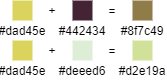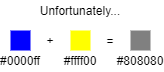（为什么蓝黄相加格外经典：因为在大部分人的生活经验里，蓝加黄就应该是绿色！相比之下红和天蓝、绿和粉红相加得到灰色，好像就没那么不可接受。）

#### Subtractive blending: CMYK

RGB 模型的乘法混合（正片叠底）解决了普通补色的混合，但依然无法解决蓝黄问题，它们相乘后会变成 #000000 也就是黑色。这并不是我们想看到的，所以否决。

CMYK 模型的蓝色 #00f 和黄色 #ff0 分别对应 (1, 1, 0, 0) 和 (0, 0, 1, 0)，CMYK 的加法运算等于 RGB 的乘法运算，故得到 (1, 1, 1, 0)，也是纯黑色。

The whole idea with RGB to CMYK conversion is to create a CMYK image which on print will look as similar as possible to the original RGB image.

A CMYK color is not an objective color, it's simply a set of technical instructions for the printing device on which halftone percentages to use.1. 先把所有的白色抠出来 $rgb_{RYB} –= min(rgb_{RGB})$
2. 从红色分量里把黄色抠出来 $r_{RYB} = r_{RGB} – min(r_{RGB}, g_{RGB})$，黄色晾干备用（因为 RGB 的黄色是红绿组成的）
3. 从绿色分量里把黄色抠出来，剩下的绿色晾干备用 $g_{RGB} – min(r_{RGB}, g_{RGB})$
4. 把这部分多余的绿色和蓝色搓在一起，形成新的蓝色分量 $b_{RYB} = (b_{RGB} + g_{RGB} – min(r_{RGB}, g_{RGB}) / 2$（因为 RGB 的绿色要拆进 RYB 的蓝和黄）
5. 把原本的绿色和之前抠出来的黄色搓在一起，形成新的黄色分量 $y_{RYB} = (g_{RGB} + min(r_{RGB}, g_{RGB}) / 2$
6. 最后，加回去一个黑色 $rgb_{RYB} += min(1 – rgb_{RGB})$

（🚧 施工中）

### Next

import java.util.*;
import java.text.*;

color brush_color = color(0, 0, 0);
float brush_alpha = 0.0;
Paint[] palette = new Paint ;

void setup() {
size(256, 256);

palette = new Paint(color(0,0,0), 1.0, 0);
palette = new Paint(color(255,255,255), 0.0, 1);

for (int i = 0; i < min(lines.length, 14); i++) {
int[] rgb = int(split(lines[i], ' '));
palette[i+2] = new Paint(color(rgb, rgb, rgb), 1.0, i+2);
}

for (int i = lines.length; i < 14; i++){
palette[i+2] = new Paint(color(255,255,255), 0.0, i+2);
}
}

void draw() {
background(128);
for (int i=0; i < palette.length; i++) {
palette[i].display();
}
}

void mousePressed(){
int idx = get_color_index(mouseX, mouseY);
if (mouseButton == LEFT) {
palette[idx].mix();
}
else if (mouseButton == RIGHT) {
palette[idx].pick();
}
}

int get_color_index(int x, int y) {
int row = y / 64;
int col = x / 64;
return row * 4 + col;
}

public class Paint {
color paint_color = color(0,0,0);
float paint_alpha = 0.0;
int index = 0;

Paint(color c, float a, int i) {
paint_color = c;
paint_alpha = a;
index = i;
}

void mix() {
float weight = constrain(brush_alpha / (paint_alpha + brush_alpha), 0.0, 1.0);
paint_color = lerpColor(paint_color, brush_color, weight);
paint_alpha = (weight + paint_alpha) / 2;
display();
}

void pick() {
brush_color = paint_color;
brush_alpha = paint_alpha;
}

void display() {
noStroke();
fill(paint_color, paint_alpha * 255);
rect(index % 4 * 64, index / 4 * 64, 64, 64);
}
}


color mixRYB(color c1, color c2, float w) {
color c1RYB = RGB2RYB(c1);
color c2RYB = RGB2RYB(c2);
color cRYB = lerpColor(c1RYB, c2RYB, w);
color c = RYB2RGB(cRYB);
return c;
}

// algorithm: http://nishitalab.org/user/UEI/publication/Sugita_SIG2015.pdf
// code: http://www.deathbysoftware.com/colors/index.html

color RGB2RYB(color rgb){
float r = red(rgb);
float g = green(rgb);
float b = blue(rgb);
float w = min(r,g,b);
r -= w;
g -= w;
b -= w;
float maxg = max(r,g,b);
float y = min(r,g);
r -= y;
g -= y;
if (b > 0 && g > 0) {
b /= 2;
g /= 2;
}
y += g;
b += g;
float maxy = max(r,y,b);
if (maxy > 0) {
float n = maxg / maxy;
r *= n;
y *= n;
b *= n;
}
color ryb = color(r+w, y+w, b+w);
return ryb;
}

color RYB2RGB(color ryb){
float r = red(ryb);
float y = green(ryb);
float b = blue(ryb);
float w = min(r,y,b);
r -= w;
y -= w;
b -= w;
float maxy = max(r,y,b);
float g = min(y,b);
y -= g;
b -= g;
if (b > 0 && g > 0) {
b *= 2;
g *= 2;
}
r += y;
g += y;
float maxg = max(r,g,b);
if (maxg > 0) {
float n = maxy / maxg;
r *= n;
g *= n;
b *= n;
}
color rgb = color(r+w, g+w, b+w);
return rgb;
}


## Processing 笔记本 (1)

### Getting Started

Getting Started \ Processing.org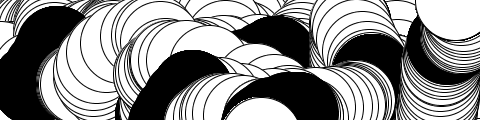import java.util.*;
import java.text.*;

void setup() {
size(480, 120);
}

void draw() {
if (mousePressed) {
fill(0);
} else {
fill(255);
}
ellipse(mouseX, mouseY, 80, 80);
}

void keyPressed() {
if (key == 's') {
DateFormat formatter = new SimpleDateFormat("yyMMddHHmmss");
Date d = new Date();
String clock = formatter.format(d);
save("ellipse_" + clock + ".png");
}
}


processing 的工程叫 sketch，下文都会用这个词代指。sketch 由多个代码片段组成。一个 sketch 会有一个专属目录，save() 函数保存的图片就放在那里面。上面这个 sketch 的保存功能还可以这么实现：

saveFrame("ellipse_####.png");


#### 是填充数字的模板，试验下来发现填充的是帧数。

### Overview

Processing Overview \ Processing.org

• setup() ：初始化方法
• size(w, h)：设置窗口分辨率，必须写在 setup() 的第一行。可以用不同的 renderer 来调用 size，例如 size(400, 400, P2D) 是 2D 图像（默认的），size(400, 400, P3D) 是 3D，size(400, 400, PDF, "output.pdf") 是写入 PDF 文件。
• width, height：当前窗口宽高
• draw()：每帧刷新
• mousePressed()：鼠标按下时事件
• mousePressed：判断鼠标是否按下的布尔值
• mouseX, mouseY：当前鼠标的坐标
• keyPressed()：按键事件

String[] lines = loadStrings("something.txt");